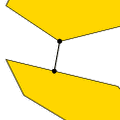CGAL 4.7 - Optimal Distances
Optimal Distances ReferenceKaspar Fischer, Bernd Gärtner, Thomas Herrmann, Michael Hoffmann, and Sven Schönherr
This package provides algorithms for computing the distance between the convex hulls of two point sets in d-dimensional space, without explicitely constructing the convex hulls. It further provides an algorithm to compute the width of a point set, and the furthest point for each vertex of a convex polygon.

Introduced in: CGAL 1.1
BibTeX: cgal:fghhs-od-15b
License: GPL

## Assertions

The optimization code uses infix OPTIMISATION in the assertions, e.g. defining the compiler flag CGAL_OPTIMISATION_NO_PRECONDITIONS switches precondition checking off, cf. Section Checks.

## All furthest neighbors

• CGAL::all_furthest_neighbors_2
• AllFurthestNeighborsTraits_2

## Width

• CGAL::Width_3<Traits>
• CGAL::Width_default_traits_3<K>
• WidthTraits_3

## Polytope Distance

• CGAL::Polytope_distance_d<Traits>
• CGAL::Polytope_distance_d_traits_2<K,ET,NT>
• CGAL::Polytope_distance_d_traits_3<K,ET,NT>
• CGAL::Polytope_distance_d_traits_d<K,ET,NT>
• PolytopeDistanceDTraits

Concepts

## Classes

class  CGAL::Polytope_distance_d< Traits >
An object of the class Polytope_distance_d represents the (squared) distance between two convex polytopes, given as the convex hulls of two finite point sets in $$d$$-dimensional Euclidean space $$\E^d$$. More...

class  CGAL::Polytope_distance_d_traits_2< K, ET, NT >
The class Polytope_distance_d_traits_2 is a traits class for the $$d$$-dimensional optimisation algorithms using the two-dimensional CGAL kernel. More...

class  CGAL::Polytope_distance_d_traits_3< K, ET, NT >
The class Polytope_distance_d_traits_3 is a traits class for the $$d$$-dimensional optimisation algorithms using the three-dimensional CGAL kernel. More...

class  CGAL::Polytope_distance_d_traits_d< K, ET, NT >
The class Polytope_distance_d_traits_d is a traits class for the $$d$$-dimensional optimisation algorithms using the $$d$$-dimensional CGAL kernel. More...

class  CGAL::Width_3< Traits >
Given a set of points $$\mathcal{S}=\left\{p_1,\ldots , p_n\right\}$$ in $$\mathbb{R}^3$$. More...

class  CGAL::Width_default_traits_3< K >
The class Width_default_traits_3 is a traits class for Width_3<Traits> using the three-dimensional CGAL kernel. More...

## Functions

template<class RandomAccessIterator , class OutputIterator , class Traits >
OutputIterator CGAL::all_furthest_neighbors_2 (RandomAccessIterator points_begin, RandomAccessIterator points_end, OutputIterator o, Traits t=Default_traits)
computes all furthest neighbors for the vertices of the convex polygon described by the range [points_begin, points_end), writes their indices (relative to points_begin) to othe furthest neighbor of points_begin[i] is points_begin[i-th number written to o] and returns the past-the-end iterator of this sequence. More...

## Function Documentation

template<class RandomAccessIterator , class OutputIterator , class Traits >
 OutputIterator CGAL::all_furthest_neighbors_2 ( RandomAccessIterator points_begin, RandomAccessIterator points_end, OutputIterator o, Traits t = Default_traits )

computes all furthest neighbors for the vertices of the convex polygon described by the range [points_begin, points_end), writes their indices (relative to points_begin) to othe furthest neighbor of points_begin[i] is points_begin[i-th number written to o] and returns the past-the-end iterator of this sequence.

The function all_furthest_neighbors_2() computes all furthest neighbors for the vertices of a convex polygon $$P$$, i.e. for each vertex $$v$$ of $$P$$ a vertex $$f_v$$ of $$P$$ such that the distance between $$v$$ and $$f_v$$ is maximized.

Precondition
The points denoted by the non-empty range [points_begin, points_end) form the boundary of a convex polygon $$P$$ (oriented clock- or counterclockwise).

The geometric types and operations to be used for the computation are specified by the traits class parameter t. This parameter can be omitted if RandomAccessIterator refers to a point type from a Kernel. In this case, the kernel is used as default traits class.

Requires

1. If t is specified explicitly, Traits is a model for AllFurthestNeighborsTraits_2.
2. Value type of RandomAccessIterator is Traits::Point_2 or, if t is not specified explicitly, K::Point_2 where K is a model for Kernel.
3. OutputIterator accepts int as value type.
See Also
AllFurthestNeighborsTraits_2
CGAL::monotone_matrix_search()

Implementation

The implementation uses monotone matrix search . Its runtime complexity is linear in the number of vertices of $$P$$.

Example

The following code generates a random convex polygon p with ten vertices, computes all furthest neighbors and writes the sequence of their indices (relative to points_begin) to cout (e.g. a sequence of 4788911224 means the furthest neighbor of points_begin is points_begin, the furthest neighbor of points_begin is points_begin etc.).

#include <CGAL/Cartesian.h>
#include <CGAL/Polygon_2.h>
#include <CGAL/point_generators_2.h>
#include <CGAL/random_convex_set_2.h>
#include <CGAL/all_furthest_neighbors_2.h>
#include <CGAL/IO/Ostream_iterator.h>
#include <iostream>
#include <vector>
typedef double FT;
typedef Kernel::Point_2 Point;
typedef std::vector<int> Index_cont;
typedef CGAL::Polygon_2<Kernel> Polygon_2;
typedef CGAL::Random_points_in_square_2<Point> Generator;
int main()
{
// generate random convex polygon:
Polygon_2 p;
CGAL::random_convex_set_2(10, std::back_inserter(p), Generator(1));
// compute all furthest neighbors:
CGAL::all_furthest_neighbors_2(p.vertices_begin(), p.vertices_end(),
Oiterator(std::cout));
std::cout << std::endl;
return 0;
}

#include <CGAL/all_furthest_neighbors_2.h>

Examples:
Polytope_distance_d/all_furthest_neighbors_2.cpp.